## How to Calculate and Solve for Total Compressibility of a Reservoir Fluid Flow | The Calculator Encyclopedia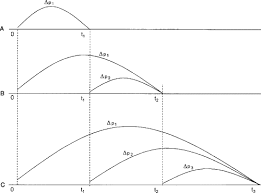The image above represents the total compressibility.

To compute for the total compressibility, seven essential parameters are needed and these parameters are Water compressibility (Cw), Oil compressibility (Co), Gas compressibility (Cg), Rock compressibility (Cr), water saturation (Sw), Oil saturation (So) and Gas saturation (Sg).

The formula for calculating the total compressibility:

CT = CoSo + CgSg + CwSw + Cr

Where;

CT = Total Compressibility
Cw = Water Compressibility
Co = Oil Compressibility
Cg = Gas Compressibility
Cr = Rock Compressibility
Sw = Water Saturation
So = Oil Saturation
Sg = Gas Saturation

Let’s solve an example;
With a given value of 13 as water compressibility, 17 as oil compressibility, 19 as gas compressibility, 25 as rock compressibility, 24 as water saturation, 29 as oil saturation and 21 as gas saturation.
Find the total compressibility?

This implies that;

Cw = Water Compressibility = 13
Co = Oil Compressibility = 17
Cg = Gas Compressibility = 19
Cr = Rock Compressibility = 25
Sw = Water Saturation = 24
So = Oil Saturation = 29
Sg = Gas Saturation = 21

CT = CoSo + CgSg + CwSw + Cr
CT = (17 x 29) + (19 x 21) + (13 x 24) + 25
CT = 493 + 399 + 312 + 25
CT = 1229

Therefore, the total compressibility is 1229 psi.

## How to Calculate and Solve for Solution Gas – Oil Ratio of a Reservoir Fluid Flow | The Calculator Encyclopedia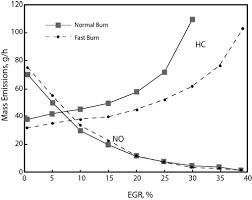The image above represents a Gas – Oil Ratio.

To compute for the gas – oil ratio, seven essential parameters are needed and these parameters are Solution Gas (Rs), Oil Viscosity (μo), Gas Viscosity (μg), Relative Permeability to Gas (krg), Relative Permeability to Oil (kro), Gas Formation Volume Factor (Bg) and Oil Formation Volume Factor (Bo).

The formula for calculating the gas – oil ratio:

GOR = Rs + [krg /  kro] [μoBo / μgBg]

Where;

GOR = Solution Gas-Oil Ratio
Rs = Solution Gas
μo = Oil Viscosity
μg = Gas Viscosity
krg = Relative Permeability to Gas
kro = Relative Permeability to Oil
Bg = Gas Formation Volume Factor
Bo = Oil Formation Volume Factor

Let’s solve an example;
Find the Gas – Oil Ratio with a solution gas of 11, oil viscosity of 7, gas viscosity of 9, relative permeability to gas of 12, relative permeability to oil of 16, gas formation volume factor of 22 and oil formation volume factor of 18.

This implies that;

Rs = Solution Gas = 11
μo = Oil Viscosity = 7
μg = Gas Viscosity = 9
krg = Relative Permeability to Gas = 12
kro = Relative Permeability to Oil = 16
Bg = Gas Formation Volume Factor = 22
Bo = Oil Formation Volume Factor = 18

GOR = Rs + [krg /  kro] [μoBo / μgBg]
GOR = 11 + [12/16] [7 x 18 /9 x 22]
GOR = 11 + [12/16] [126/198]
GOR = 11 + [12/16] [0.63]
GOR = 11 + [0.75] [0.63]
GOR = 11 + 0.477
GOR = 11.477

Therefore, the Gas – Oil Ratio is 11.477.

## How to Calculate and Solve for the Water – Oil Ratio of a Reservoir Fluid Flow | The Calculator Encyclopedia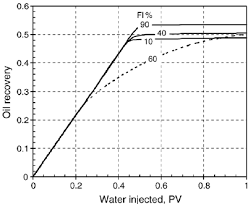The above image represents Water – Oil Ratio.

To compute for the water – oil ratio, six essential parameters are needed and these parameters are o)Oil Viscosity, (μw)Water Viscosity, (krw)Relative Permeability to Water, (kro)Relative Permeability to Oil, (Bw)Water Formation Volume Factor and (Bo)Oil Formation Volume Factor.

The formula for calculating the water – oil ratio:

WOR = [krw / kro] [μoBo  / μwBw ]

Where;

WOR = Water Oil Ratio
μo = Oil Viscosity
μw = Water Viscosity
krw = Relative Permeability to Water
kro = Relative Permeability to Oil
Bw = Water Formation Volume Factor
Bo = Oil Formation Volume Factor

Let’s solve an example;
Find the water – oil ratio when the oil viscosity is 9, water viscosity is 11 with a relative permeability to water of 21, relative permeability to oil of 7 and a water formation volume factor of 14 with oil formation volume factor of 27.

This implies that;

μo = Oil Viscosity = 9
μw = Water Viscosity = 11
krw = Relative Permeability to Water = 21
kro = Relative Permeability to Oil = 7
Bw = Water Formation Volume Factor = 14
Bo = Oil Formation Volume Factor = 27

WOR = [krw / kro] [μoBo  / μwBw ]
WOR = [21/7] [9 x 27 /11 x 14]
WOR = [21/7] [243/154]
WOR = [21/7] [1.5779]
WOR =  [1.5779]
WOR = 4.73

Therefore, the Water – Oil Ratio is 4.73.

## How to Calculate and Solve for the Average Pressure of a Reservoir Fluid Flow | The Calculator Encyclopedia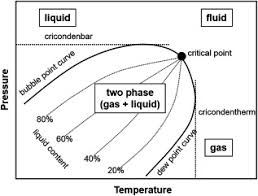The above image represents the reservoir fluid.

To compute for the reservoir fluid, two essential parameters are needed and these parameters are External pressure (Peand Flowing Bottom-Hole Pressure (Pwf).

The formula for calculating the average pressure of the reservoir fluid:

P* = √[Pe² + Pwf² / 2]

Where;

P* = Average Pressure
Pe = External Pressure
Pwf = Flowing Bottom-Hole Pressure

Let’s solve an example;
Find the average pressure of a reservoir fluid with an external pressure of 19 and Flowing bottom-hole pressure is 15.

This implies that;

Pe = External Pressure = 19
Pwf = Flowing Bottom-Hole Pressure = 15

P* = √[Pe² + Pwf² / 2]
P* = √[19² + 15² / 2]
P* = √[361 + 225 / 2]
P* = √[586 / 2]
P* = √
P* = 17.117

Therefore, the average pressure is 17.117 psi;.

Calculating the External Pressure when the Average Pressure and the Flowing Button-Hole Pressure is Given.

Pe = √[[P* x 2]² – Pwf²]

Where;

Pe = External Pressure
P* = Average Pressure
Pwf = Flowing Bottom-Hole Pressure

Let’s solve an example;
Find the external pressure of a reservoir fluid with an average pressure of 24 and Flowing bottom-hole pressure is 16.

This implies that;

P* = Average Pressure = 24
Pwf = Flowing Bottom-Hole Pressure = 16

Pe = √[[P* x 2]² – Pwf²]
Pe = √[[24 x 2]² – 16²]
Pe = √
Pe = 45.25

Therefore, the external pressure is 45.25.

## How to Calculate and Solve for a Well Drainage Area | Nickzom Calculator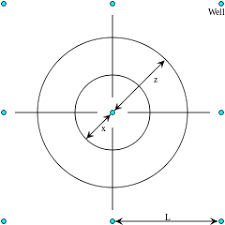The image above represents well drainage area.

To compute for the well drainage area, three essential param xeters are needed and these parameters are Total Area of Field (AT), Well Flow Rate (qwand Field Flow Rate (qr).

The formula for calculating the well drainage area:

Aw = Ar[qw / qr]

Where;

Aw = Well Drainage Area
AT = Total Area of Field
qw = Well Flow Rate
qr = Field Flow Rate

Let’s solve an example;
Find the well drainage area when the total area of field is 11, well flow rate is 9 and the field flow rate is 6.

This implies that;

AT = Total Area of Field = 11
qw = Well Flow Rate = 9
qr = Field Flow Rate = 6

Aw = AT[qw / qr]
Aw = 11[9/6]
Aw = 11[1.5]
Aw = 16.5

Therefore, the well drainage area is 16.5 ft².

Calculating the Total Area of Field when Well Drainage Area, Well Flow Rate and Field Flow Rate is Given.

AT = Aw (qr / qw)

Where;
AT = Total Area of Field
Aw = Well Drainage Area
qw = Well Flow Rate
qr = Field Flow Rate

Let’s solve an example;
Find the total area of field when the well drainage area is 32, well flow rate is 11 and the field flow rate is 14.

This implies that;
Aw = Well Drainage Area = 32
qw = Well Flow Rate = 11
qr = Field Flow Rate = 14

AT = Aw (qr / qw)
AT = 32 (14 / 11)
AT = 32 (1.272)
AT = 40.704

Therefore, the total area of field is 40.704.

Calculating the Field Flow Rate when Well Drainage Area, Total Area of Field and Well Flow Rate is Given.

qr = qw (Ar / Aw)

Where;
qr = Field Flow Rate
AT = Total Area of Field
Aw = Well Drainage Area
qw = Well Flow Rate

Let’s solve an example;
Find the field flow rate when the well drainage area is 42, total area of field is 32 and the well flow rate is 24.

This implies that;
Aw = Well Drainage Area = 42
AT = Total Area of Field = 32
qw = Well Flow Rate = 24

qr = qw (AT / Aw)
qr = 24 (32 / 42)
qr = 24 (0.7619)
qr = 18.285

Therefore, the Field Flow Rate is 18.285.

## RE: Passion, Purpose and DutyOn a bright Saturday afternoon of November, 2013 I had just finished doing my chores. I was very tired and just wanted to relax with my very entertaining soccer video game. Yes, I am a FIFA guy, FIFA 14 was hot and I was killing the tournament. I was at the finals of the tournament and there was so much pride, joy and contentment at the level I reached on the very tough game.

As I was about to start the finals match, I heard a knock on my door. Behold, it was my younger sister. She always came to me to help her solve her mathematics problems or assignments. She always complained that the teachers are doing magic when it comes to solving math and she gets lost in the steps. I noticed that after a while of helping her that the teachers do skip some steps when solving the math problem assuming that the students know them but alas most do not.

I have already started the game and I decided to joggle the both activities since the math problem she brought was on quadratic equation which is very simple for me as a second year engineering student. In the process of helping her understand how to use the almighty quadratic formula I was scored twice before half time and at half time I was able to have come back with a goal making it 1–2 (Me Versus Computer). I still pressed forward to the next half and along the way I was scored again and I was so angry. I ignored my sister to focus on just the game to know if I could come back. But I was only able to come back with a goal and the finals ended as 2–3 with me losing the tournament. I was so pissed off. I yelled! My sister on the hand was sad I did not aid her understand how to solve her math problem. She left the room unsatisfied.

In the midst of my vex I wished in my mind that there was something like a calculator encyclopedia that she can always use whenever she has a math problem just like a dictionary. No one would disturb you to tell you the meaning of a word. You simply tell them to go and check their dictionary and that’s all. I thought why is there nothing like that for calculations? Continue reading RE: Passion, Purpose and Duty